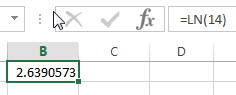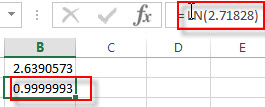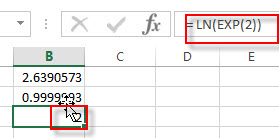# Excel LN Function

This post will guide you how to use Excel LN function with syntax and examples in Microsoft excel.

### Description

The Excel LN function returns the natural logarithm of a given number.  And the natural logarithms are based on the constant e (the value is equal to 2.718281828…).

The LN function is a build-in function in Microsoft Excel and it is categorized as a Math and Trigonometry Function.

The LN function is available in Excel 2016, Excel 2013, Excel 2010, Excel 2007, Excel 2003, Excel XP, Excel 2000, Excel 2011 for Mac.

### Syntax

The syntax of the LN function is as below:

`= LN (number)`

Where the LN function arguments is:

• number – This is a required argument. The number that you want the logarithm. And the number must be a positive real number.

Note:

• If either the supplied number argument is negative or zero, the LN function will return #NUM! Error.
• If the number argument is non-numeric, the LN function will return #VALUE! Error.
• LN is the inverse of the Exp function.

### Excel LN Function Examples

The below examples will show you how to use Excel LN Function to calculate the natural logarithm of a given number.

1# to get the natural logarithm of 14, enter the following formula in Cell B1.

`= LN (14)`2# to get the natural logarithm of the value of the constant e (2.71828), enter the following formula in Cell B2.

`= LN (2.71828)`3# to get the natural logarithm of e raised to the power 2, enter the following formula in Cell B2.

`= LN (EXP(2))`### Related Functions

• Excel LOG10 function
The Excel LOG10 function returns the base-10 logarithm of a given number.The syntax of the LOG10 function is as below:= LOG10 (number)…
• Excel LOG function
The Excel LOG function returns the logarithm of a given number based on a supplied base. And the default base argument is 10.The syntax of the LOG function is as below:= LOG (number,[base])…
Related Posts

Excel DVAR Function

This post will guide you how to use Excel DVAR function with syntax and examples in Microsoft excel. Description The Excel DVAR Function will get the variance of a population based on a sample of numbers in a column in ...

Excel DSUM Function

This post will guide you how to use Excel DSUM function with syntax and examples in Microsoft excel. Description The Excel DSUM Function will add the numbers in a column or database that meets a given criteria. And so you ...

Excel DSTDEVP Function

This post will guide you how to use Excel DSTDEVP function with syntax and examples in Microsoft excel. Description The Excel DSTDEVP returns the standard deviation of a population based on the entire population of numbers in a column or ...

Excel DSTDEV Function

This post will guide you how to use Excel DSTDEV function with syntax and examples in Microsoft excel. Description The Excel DSTDEV returns the standard deviation of a population based on a sample of numbers in a column or database ...

Excel DMIN Function

This post will guide you how to use Excel DMIN function with syntax and examples in Microsoft excel. Description The Excel DMIN returns the minimum value from a database or a column of a list that matches the specified conditions. ...

Excel DPRODUCT Function

This post will guide you how to use Excel DPRODUCT function with syntax and examples in Microsoft excel. Description The Excel DPRODUCT returns the product of values from a set of records that match criteria that you specify. The DPRODUCT ...

Excel XIRR Function

This post will guide you how to use Excel XIRR function with syntax and examples in Microsoft excel. Description The Excel XIRR function returns the internal rate of return for a series of cash flows that is not necessarily periodic. ...

Excel VDB Function

This post will guide you how to use Excel VDB function with syntax and examples in Microsoft excel. Description The Excel VDB function calculates the depreciation of an asset for a specified period based on the double declining balance method. ...

Excel SYD Function

This post will guide you how to use Excel SYD function with syntax and examples in Microsoft excel. Description The Excel SYD function calculates the sum-of-years’digits depreciation of an asset for a specified period. And it will return a numeric ...

Excel SLN Function

This post will guide you how to use Excel SLN function with syntax and examples in Microsoft excel. Description The Excel SLN function calculates the depreciation of an asset for one period based on the straight line depreciation. And it ...

Sidebar